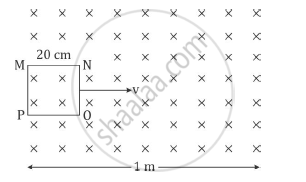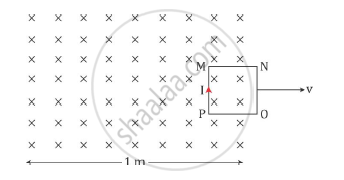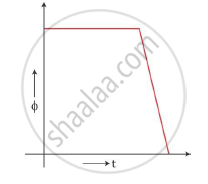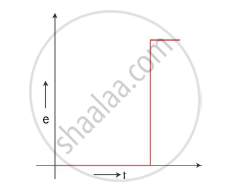# Plot a Graph Showing the Variation of Magnetic Flux and Induced Emf as a Function of Time. - Physics

Sum

A square loop MNOP of side 20 cm is placed horizontally in a uniform magnetic field acting vertically downwards as shown in the figure. The loop is pulled with a constant velocity of 20 cm s−1 till it goes out of the field.(i) Depict the direction of the induced current in the loop as it goes out of the field. For how long would the current in the loop persist?

(ii) Plot a graph showing the variation of magnetic flux and induced emf as a function of time.

#### Solution

(i)The direction of induced current in the loop as it goes out is depicted in the figure below.The current will persist till the entire loop comes out of the field. Hence, we have

t=d/v=(20 cm)/(20

Hence, the current will persist for 1 second.

(ii)The magnetic flux in the coil when it is inside the field is constant. This maximum flux is given as Φ = Bla (a is the side of the square loop). This flux will start dropping once the loop comes out of the field and will be zero when it is completely out of the field.

The e.m.f. induced in the coil when it is inside the field is zero as the flux is not changing. When the loop just comes out of the field, the flux change is maximum and the e.m.f. induced is e=(dphi)/dt=-Bl(db)/(dt)=-Blv This e.m.f remains constant till the entire loop comes out. When the loop is completely out of the field, the e.m.f. drops to zero again.Concept: Magnetic Flux
Is there an error in this question or solution?
2014-2015 (March) Panchkula Set 3

Share